# Math in Focus Grade 2 Chapter 15 Practice 2 Answer Key Multiplying 3: Using Dot Paper

This handy Math in Focus Grade 2 Workbook Answer Key Chapter 15 Practice 2 Multiplying 3: Using Dot Paper detailed solutions for the textbook questions.

## Math in Focus Grade 2 Chapter 15 Practice 2 Answer Key Multiplying 3: Using Dot Paper

Use dot paper to solve.

Example
Sally buys 4 lanterns. Each lantern costs $3. How much does Sally pay for the lanterns?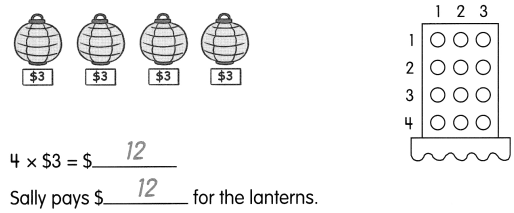Question 1. Nicole buys 6 soup bowls. Each soup bowl costs$3. How much does she pay for all the soup bowls?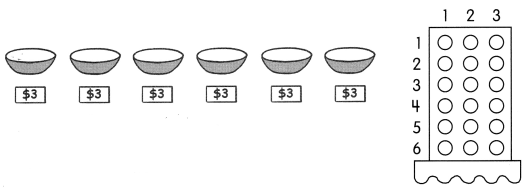___________ × $3 =$___________
She pays $__________ for all the soup bowls. Answer: 6 x$3 = $18 She pays$18 for all the soup bowls.

Use dot paper to solve.

Question 2.
There are 7 tricycles. Each tricycle has 3 wheels. How many wheels are there in all?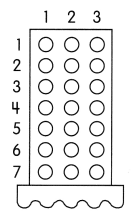___________ × ___________ = ___________
There are ___________ wheels in all.
7 x 3 = 21
There are 21 wheels in all.

Question 3.
There are 8 groups of children in the class. There are 3 children in each group. How many children are there in the class?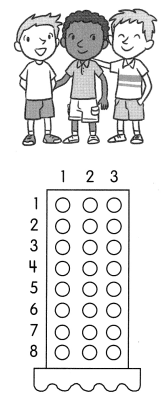___________ × ___________ = ___________
There are ___________ children in the class.
8 x 3 = 24
There are 24 children in the class.

Example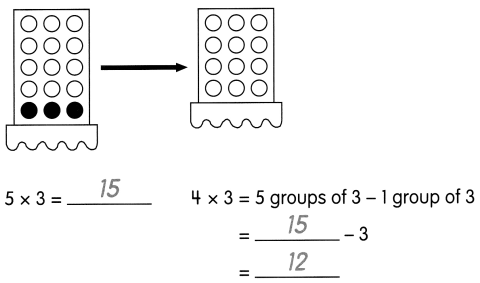Question 4.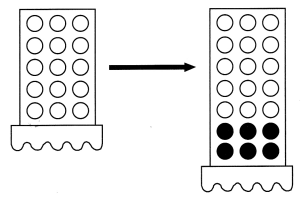5 × 3 = ____________
7 × 3 = 5 groups of 3 + __________ groups of 3
= __________ + ___________
= ___________
5 × 3 = 15
7 × 3 = 5 groups of 3 + 2 groups of 3
= 15 + 6
= 21.

Question 5.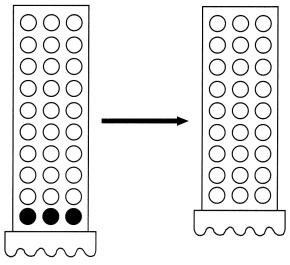10 × 3 = ____________
9 × 3 = 10 groups of 3 – __________ groups of 3
= __________ – ___________
= ___________
10 × 3 = 30
9 × 3 = 10 groups of 3 – 1 groups of 3
= 30 – 3
= 27.

Question 6.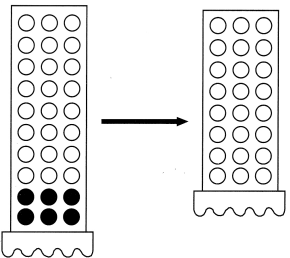10 × 3 = ____________
8 × 3 = 10 groups of 3 – __________ groups of 3
= __________ – ___________
= ___________
10 × 3 = 30
8 × 3 = 10 groups of 3 – 2 groups of 3
= 30 – 6
= 24.

Example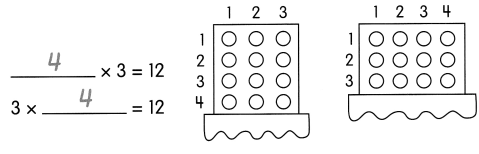Question 7.
___________ × 3 = 18
3 × __________ = 18
6 x 3 =  18
3 x 6 = 18.

Question 8.
___________ × 3 = 21
3 × __________ = 21
7 x 3 = 21
3 x 7 = 21

Question 9.
8 × 3 = __________
3 × 8 = __________